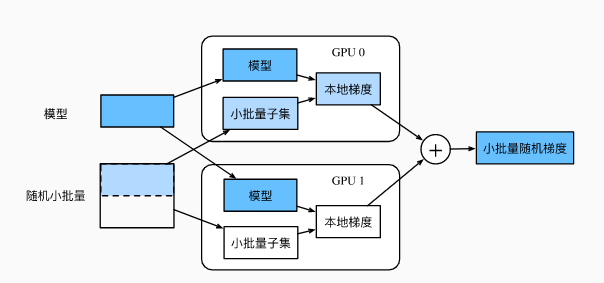# 『开发技术』GPU训练加速原理（附KerasGPU训练技巧）

## 0.深入理解GPU训练加速原理

GPU是如何加速的呢？

• 单个GPU较于CPU加速：

• 多GPU较于单GPU加速：使用2块GPU的数据并行下的小批量随机梯度的计算

## 1.如何在 GPU 上运行 Keras?

THEANO_FLAGS=device=gpu,floatX=float32 python my_keras_script.py"gpu" 可能需要根据你的设备标识符（例如gpu0，gpu1等）进行更改。

import theano
theano.config.device = 'gpu'
theano.config.floatX = 'float32'## 2.如何在多 GPU 上运行 Keras 模型?

from keras.utils import multi_gpu_model

# 将 model 复制到 8 个 GPU 上。
# 假定你的机器有 8 个可用的 GPU。
parallel_model = multi_gpu_model(model, gpus=8)
parallel_model.compile(loss='categorical_crossentropy',
optimizer='rmsprop')

# 这个 fit 调用将分布在 8 个 GPU 上。
# 由于 batch size 为 256，每个 GPU 将处理 32 个样本。
parallel_model.fit(x, y, epochs=20, batch_size=256)# 模型中共享的 LSTM 用于并行编码两个不同的序列
input_a = keras.Input(shape=(140, 256))
input_b = keras.Input(shape=(140, 256))

shared_lstm = keras.layers.LSTM(64)

# 在一个 GPU 上处理第一个序列
with tf.device_scope('/gpu:0'):
encoded_a = shared_lstm(tweet_a)
# 在另一个 GPU上 处理下一个序列
with tf.device_scope('/gpu:1'):
encoded_b = shared_lstm(tweet_b)

# 在 CPU 上连接结果
with tf.device_scope('/cpu:0'):
merged_vector = keras.layers.concatenate([encoded_a, encoded_b],
axis=-1)## 3.参考

posted @ 2019-08-13 16:11  小宋是呢  阅读(1454)  评论(0编辑  收藏